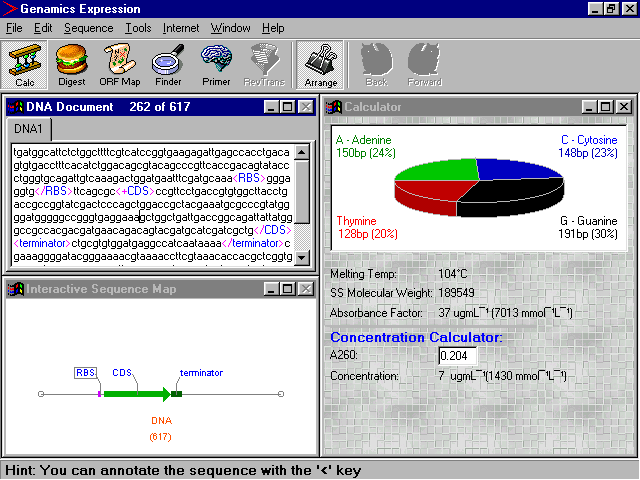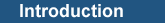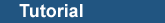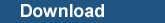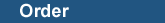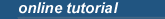Home |  JournalSeek |  SoftwareSeek |  GenomeSeek |  Expression |  Developer |  TakeOnItProperties Calculator for DNA and Proteins The Calculator Tool provides data on a variety of properties of either your protein or DNA sequences. The results are presented in an easy-to-read format. This section outlines how to use the tool, and how the various data is computed. DNA/RNA Properties The data in the Calculator window apply to either the currently selected region of the sequence, or the whole sequence if nothing is selected. The various functions are outlined below. Nucleotide Proportions The pie chart represents the proportions of the four different bases (A, T, C and G for DNA, A, U, C and G for RNA). Ambiguous characters are treated as fractions of bases, for example an R is counted as 0.5 A and 0.5 G. Melting Temperature The melting temperature of the double stranded molecule is calculated using the nearest-neighbour method for sequences less than 50 bp and the long probe method for sequences which are longer. Refer to the Primer Design tutorial for formulas for these methods. SS Molecular weight The SS (single-stranded) molecular weight is calculated for the phosphorylated, protonated form of the molecule. The following formula is used: MW = (nA x 313.21) + (nT x 288.20) + (nG x 329.21) + (nC x 289.19) + 18.02 The molecular weight of ambiguous characters are calculated as the average of its constituents, for example R is calculated as (mwA + mwG) / 2. Absorbance Factor The absorbance factor is the conversion factor used to calculate the concentration of oligonucleotides or single stranded DNA, using the measured absorbance at 260 nm (with a 1 cm lightpath). For sequences longer than 100 bp, a fixed value of 37 mmol-1cm-1 is used. For sequences less than 100 bp in length, the absorbance factor, in molL-1, is calculated using the formula: Absorbance Factor = (1 / Extinction Coefficient260) * Distance of Lightpath The extinction coefficient is computed according to Fasman (1975). The Absorbance Factor in ug/mL is then calculated using the molecular weight of the sequence (see above). The formula used is: Absorbance Factor (in ugmL-1) = Absorbance Factor (mmolL-1) / MW (gmol-1 x 103) The concentration of oligonucleotide in your solution can then be calculated by simply entering the measured A260 in the concentration calculator box. The concentration of oligonucleotide in your solution is then calculated by: Oligo Conc = Absorbance Factor x A260 Protein Properties Amino Acid Proportions The main table shows the proportions of each of the 20 amino acids, and any stop signals, in the current protein or peptide sequence. The results are shown both as an absolute count and a percentage. As with the other tabulated results in Expression, the column headings can be clicked on to sort the data in that column. Molecular Weight The molecular weight of the protein or peptide is shown in Daltons. The formula used is: MW = (nA x 71.07) + (nR x 156.18) + (nN x 114.08) + (nD x 115.08) + (nC x 103.10) + (nQ x 128.13) + (nE x 129.11) + (nG x 57.05) + (nH x 137.14) + (nI x 113.15) + (nL x 113.15) + (nK x 128.17) + (nM x 131.19) + (nF x 147.17) + (nP x 97.11) + (nS x 87.07) + (nT x 101.10) + (nW x 186.20) + (nY x 163.17) + (nV x 99.13) + 18.02 Absorbance Factor The absorbance factor is the conversion factor used to calculate the concentration of protein, using the measured absorbance at 280 nm (with a 1 cm lightpath). The following formula is used (Pace et al., 1995): Abs = (nW x 5500 + nY x 1490 + nC x 125) / MW pI This function has not yet been implemented References Fasman, GD (1975). The Handbook of Biochemistry and Molecular Biology, CRC Press, 1:589. Pace CN, Vajdos F, Fee L, Grimsley G, and Gray T (1995). How to measure and predict the molar absorption coefficient of a protein. Protein Sci, 4(10):2411-2423. Related Articles Using the Sequence Map Annotating Your Sequences Return to Expression Overview